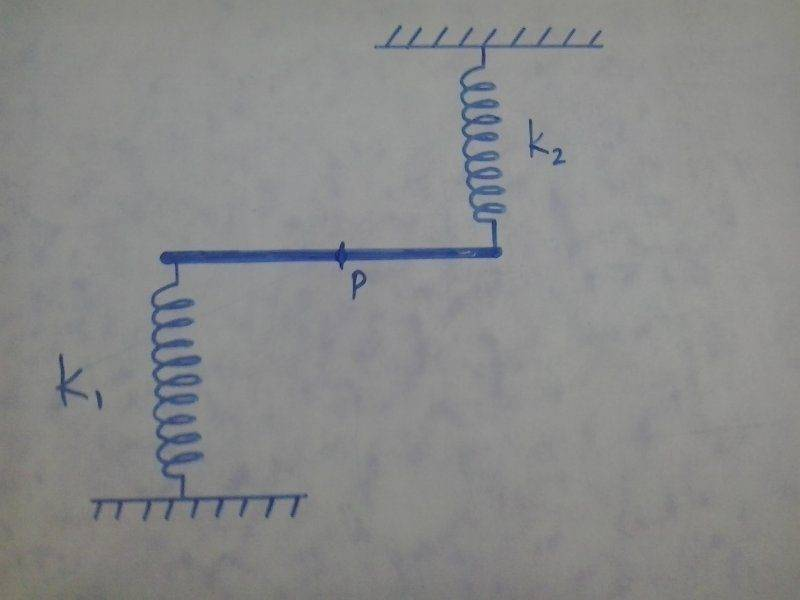# Period of oscillation

• Nathanael

Homework Helper

## Homework Statement[/B]
A system consisting of a rod of mass M and length L is pivoted at its centre P. Two springs of spring constants k1 and k2 are attached as shown. They are relaxed when the rod is horizontal. What is the time period of the rod if it is given a slight angular displacement.

## Homework Equations

M = 200
k1 = 20
k2 = 2
Presumably all in SI units

## The Attempt at a Solution

I would describe the situation by the equation
$(\frac{mL^2}{12})\frac{d^2\theta}{dt^2}=-\frac{L^2}{4}(k_1+k_2)\theta$
Because $\frac{mL^2}{12}$ is the rotational inertia, and $\frac{L^2}{4}(k_1+k_2)\theta$ is (approximately) the torque.

We don't care about the angle at time zero, so we don't need the most general solution (all solutions should have the same period) we just need a particular solution, which could be:

$\theta_{max}\sin(\sqrt{\frac{3(k_1+k_2)}{m}}t)=\theta_{max}\sin(\sqrt{\frac{66}{200}}t)$

The period should then be $T=2\pi \sqrt{\frac{100}{33}}\approx 10.9$ seconds

But the answer is apparently 6.62 secondsYour work looks OK to me. It's a little odd that the spring constants differ by so much. You would get 6.62 s if k2 = 40 rather than 2.

•Orodruin
I got the samer as you.

Your work looks OK to me.
I got the samer as you.
Okay, thank you both
It's a little odd that the spring constants differ by so much. You would get 6.62 s if k2 = 40 rather than 2.
I actually made a typo... The "correct" answer was supposed to be 0.662 seconds! Those must be some stiff springs!

You would get 6.62 s if k2 = 40 rather than 2.

So perhaps a k1 missing from the definition? k2 = 2k1?
The factor of 10 could be accounted for by having the spring constants in units of N/cm instead of N/m.

So perhaps a k1 missing from the definition? k2 = 2k1?
The factor of 10 could be accounted for by having the spring constants in units of N/cm instead of N/m.
Yes, that could be it.

So perhaps a k1 missing from the definition? k2 = 2k1?
The factor of 10 could be accounted for by having the spring constants in units of N/cm instead of N/m.
The problem states "$M=10k_1=100k_2=200$" (which bothers me because N/m does not equal Kg!)
I think the problem creator just made some mistakes.

The problem states "$M=10k_1=100k_2=200$" (which bothers me because N/m does not equal Kg!)
I think the problem creator just made some mistakes.
Yes. It's poor form for an instructor to state numbers without the attendant units. You did the best you could by assuming all SI. But the instructor had no cause to write M = 10k1 etc. as you rightly point out.

Yes. It's poor form for an instructor to state numbers without the attendant units. You did the best you could by assuming all SI. But the instructor had no cause to write M = 10k1 etc. as you rightly point out.
Sometimes I have seen problems stated as: "Assume the spring constants are k1 N/m and k2 N/m, respectively, and that the mass is M kg." With this formulation, the symbols are merely numbers but, unless you use reasonable units, conversion factors may appear in your equations ...